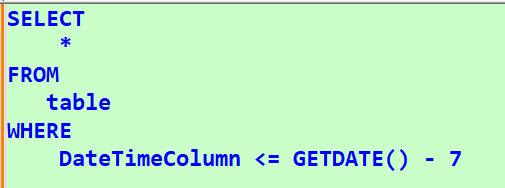# Query last 7 days on Unix timestamp column

Hello,

We have an application (CA Service Management 17.3), that uses Unix timestamps to record time. I want our Database Administrators to setup a query against this database that runs every monday, and returns the results for the last 7 days. Does anyone know how I could query against the Unix timestamp column in this working fixed example [mdb].[dbo].[act_log].[system_time], so it obtains the current timestamp minus 7 days?

Thanks
Stuart

/*
Search for all activity log entries by the analyst/contact_uid
Epoch Converter

1679223600 = Monday, 20 March 2023 00:00:00 GMT+13:00 DST
1680087600 = Thursday, 30 March 2023 00:00:00 GMT+13:00

Count of requests updated */

SELECT
COUNT (DISTINCT [mdb].[dbo].[call_req].[ref_num])
FROM [mdb].[dbo].[call_req]
INNER JOIN [mdb].[dbo].[act_log] ON [mdb].[dbo].[call_req].[persid]=[mdb].[dbo].[act_log].[call_req_id]
WHERE [mdb].[dbo].[act_log].[analyst]=0xD18B4835EA28A643800260AA1C282A87
AND [mdb].[dbo].[act_log].[system_time] > 1679223600 AND [mdb].[dbo].[act_log].[system_time] < 1680087600
AND [mdb].[dbo].[call_req].[type]='R'Maybe:

``````SELECT COUNT(DISTINCT C.ref_num) AS ref_num_count
FROM mdb.dbo.call_req C
JOIN mdb.dbo.act_log L
ON C.persid = L.call_req_id
WHERE L.analyst = 0xD18B4835EA28A643800260AA1C282A87
AND C.[type]='R'
AND L.[system_time] >= DATEDIFF(second, '1970', DATEADD(day, -7, GETUTCDATE()))
AND L.[system_time] <  DATEDIFF(second, '1970', GETUTCDATE());``````
1 Like

Hi thanks for the replies, fyi found this one works too:

SELECT
COUNT (DISTINCT [mdb].[dbo].[call_req].[ref_num])
FROM [mdb].[dbo].[call_req]
INNER JOIN [mdb].[dbo].[act_log] ON [mdb].[dbo].[call_req].[persid]=[mdb].[dbo].[act_log].[call_req_id]
WHERE [mdb].[dbo].[act_log].[analyst]=0xD18B4835EA28A643800260AA1C282A87
AND [mdb].[dbo].[act_log].[system_time] > DATEDIFF(S, '19700101 00:00:00:000', CONVERT(DateTime, DATEDIFF(DAY, +7, GETDATE())))
AND [mdb].[dbo].[call_req].[type]='R'